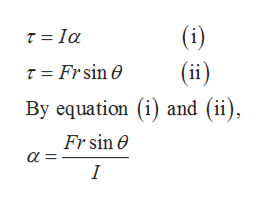# An object is acted on by a single nonzero force of magnitudeF. (a) Is it possible for the object to have zero acceleration a?(b) Is it possible for the object to have zero angular acceleration∝? (c) Is it possible for the object to be in mechanicalequilibrium?

Question
12 views

An object is acted on by a single nonzero force of magnitude
F. (a) Is it possible for the object to have zero acceleration a?
(b) Is it possible for the object to have zero angular acceleration
∝? (c) Is it possible for the object to be in mechanical
equilibrium?

check_circle

Step 1

Part (a):

Force acting on any object can be given by Newton’s second law as follows,

Step 2

From the above equation of Newton’s second law we can see that when a non-zero force is acting then acceleration of object cannot be zero.

Step 3

Part (b):

Angular acceleration of an...help_outlineImage Transcriptionclose(i) T = Ia T = Frsin O (ii) By equation (i) and (ii), Fr sin e α fullscreen

### Want to see the full answer?

See Solution

#### Want to see this answer and more?

Solutions are written by subject experts who are available 24/7. Questions are typically answered within 1 hour.*

See Solution
*Response times may vary by subject and question.
Tagged in

### Newtons Laws of Motion